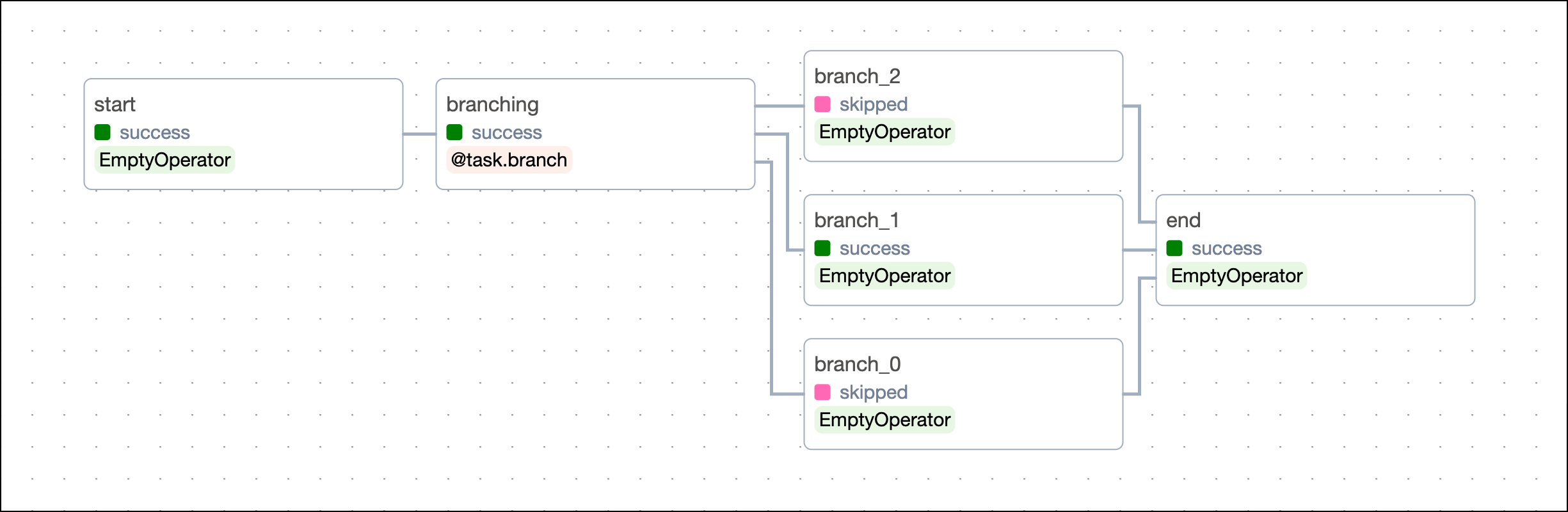Dependencies are a powerful and popular Airflow feature. In Airflow, your pipelines are defined as Directed Acyclic Graphs (DAGs). Each task is a node in the graph and dependencies are the directed edges that determine how to move through the graph. Because of this, dependencies are key to following data engineering best practices because they help you define flexible pipelines with atomic tasks.

Throughout this guide, the following terms are used to describe task dependencies:

• Upstream task: A task that must reach a specified state before a dependent task can run.
• Downstream task: A dependent task that cannot run until an upstream task reaches a specified state.

In this guide you'll learn about the many ways you can implement dependencies in Airflow, including:

• Dependency functions.
• Dynamic dependencies.
• Dependencies with the TaskFlow API.
• Trigger rules.

To view a video presentation of these concepts, see Manage Dependencies Between Airflow Deployments, DAGs, and Tasks.

The focus of this guide is dependencies between tasks in the same DAG. If you need to implement dependencies between DAGs, see Cross-DAG dependencies.

## Assumed knowledge​

To get the most out of this guide, you should have an understanding of:

## Basic dependencies​

Basic dependencies between Airflow tasks can be set in the following ways:

• Using bit-shift operators (`<<` and `>>`)
• Using the `set_upstream` and `set_downstream` methods

For example, if you have a DAG with four sequential tasks, the dependencies can be set in four ways:

• Using `set_downstream()`:

``t0.set_downstream(t1)t1.set_downstream(t2)t2.set_downstream(t3)``
• Using `set_upstream()`:

``t3.set_upstream(t2)t2.set_upstream(t1)t1.set_upstream(t0)``
• Using `>>`:

``t0 >> t1 >> t2 >> t3``
• Using `<<`:

``t3 << t2 << t1 << t0``

All of these methods are equivalent and result in the DAG shown in the following image: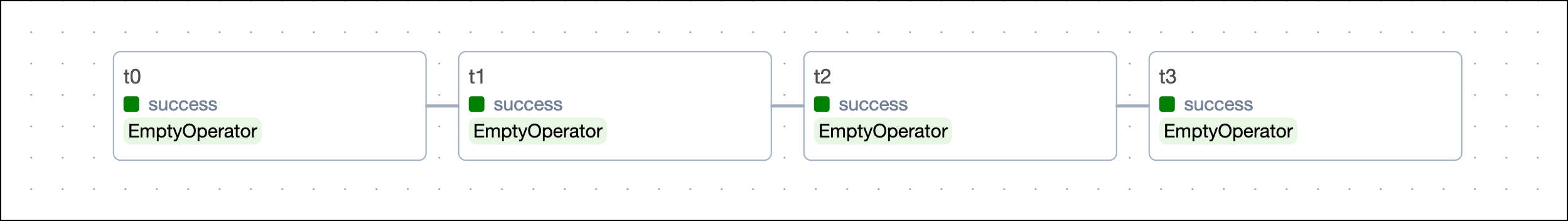Astronomer recommends using a single method consistently. Using both bit-shift operators and `set_upstream`/`set_downstream` in your DAGs can overly-complicate your code.

To set a dependency where two downstream tasks are dependent on the same upstream task, use lists or tuples. For example:

``# Dependencies with listst0 >> t1 >> [t2, t3]# Dependencies with tuplest0 >> t1 >> (t2, t3)``

These statements are equivalent and result in the DAG shown in the following image: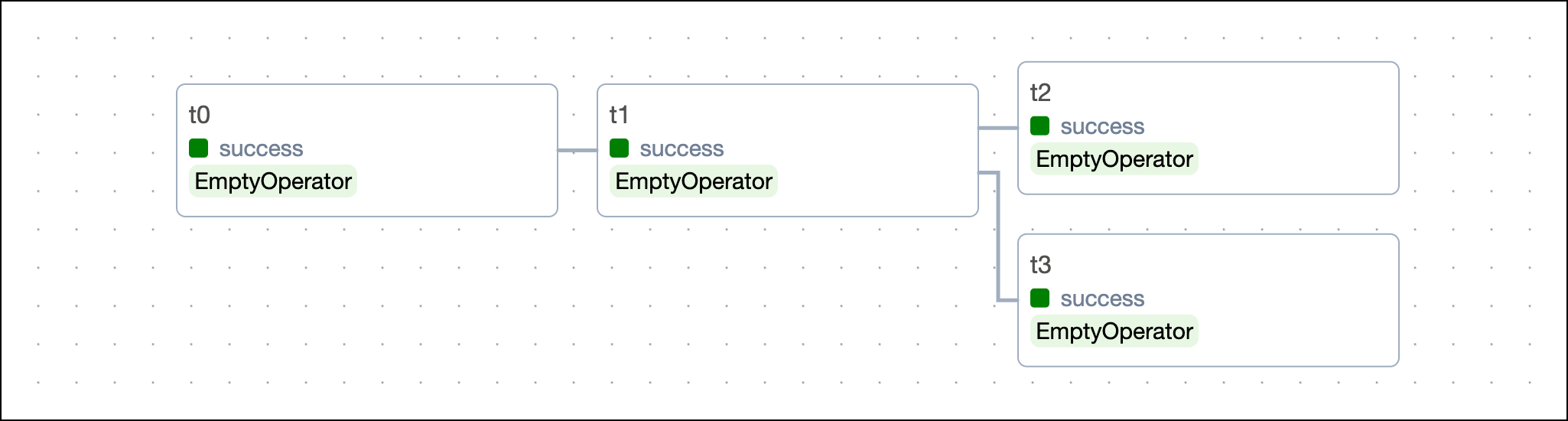When you use bit-shift operators and the `.set_upstream` and `.set_downstream` method, you can't set dependencies between two lists. For example, `[t0, t1] >> [t2, t3]` returns an error. To set dependencies between lists, use the dependency functions described in the following section.

## Dependency functions​

Dependency functions are utilities that let you set dependencies between several tasks or lists of tasks. A common reason to use dependency functions over bit-shift operators is to create dependencies for tasks that were created in a loop and are stored in a list.

``from airflow.models.baseoperator import chainlist_of_tasks = []for i in range(5):    if i % 3 == 0:        ta = EmptyOperator(task_id=f"ta_{i}")        list_of_tasks.append(ta)    else:        ta = EmptyOperator(task_id=f"ta_{i}")        tb = EmptyOperator(task_id=f"tb_{i}")        tc = EmptyOperator(task_id=f"tc_{i}")        list_of_tasks.append([ta, tb, tc])chain(*list_of_tasks)``

This code creates the following DAG structure: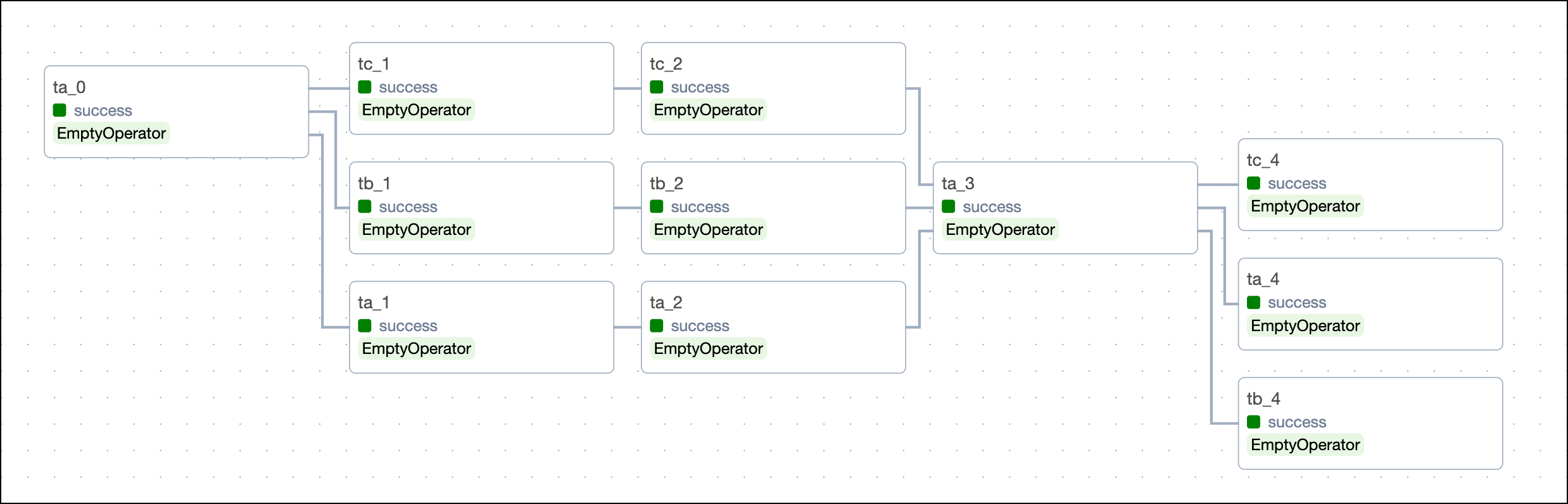### Using `chain()`​

To set parallel dependencies between tasks and lists of tasks of the same length, use the `chain()` function. For example:

``# from airflow.models.baseoperator import chainchain(t0, t1, [t2, t3, t4], [t5, t6, t7], t8)``

This code creates the following DAG structure: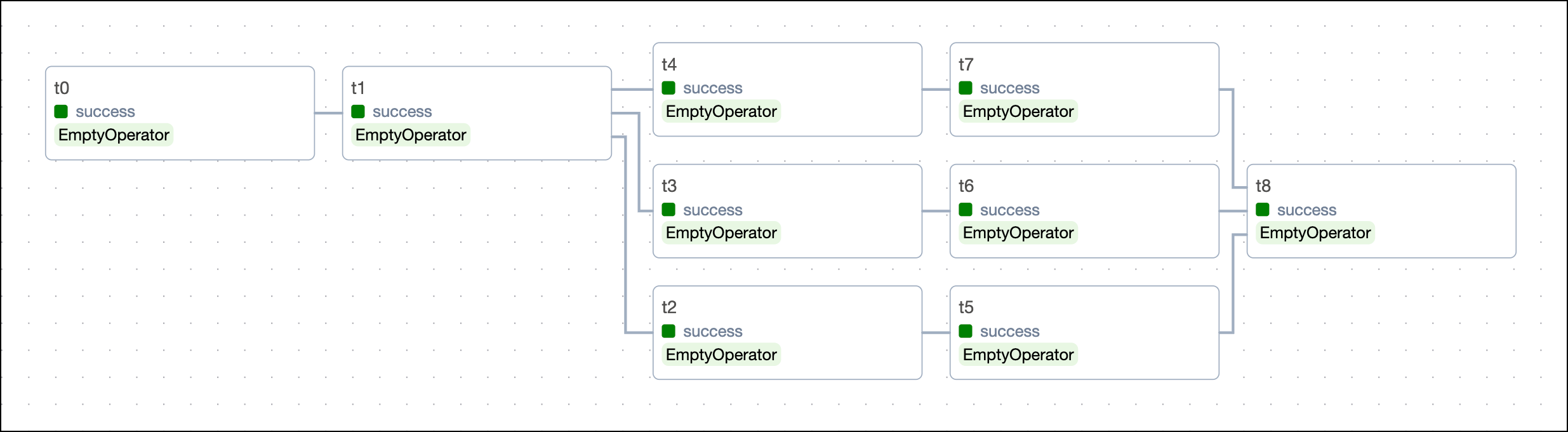When you use the `chain` function, any lists or tuples that are set to depend directly on each other need to be the same length.

``chain([t0, t1], [t2, t3])  # this code will workchain([t0, t1], [t2, t3, t4])  # this code will cause an errorchain([t0, t1], t2, [t3, t4, t5])  # this code will work``

### Using `chain_linear()`​

To set interconnected dependencies between tasks and lists of tasks, use the `chain_linear()` function. This function is available in Airflow 2.7+, in older versions of Airflow you can set similar dependencies between two lists at a time using the `cross_downstream()` function.

Replacing `chain` in the previous example with `chain_linear` creates dependencies where each element in the downstream list will depend on each element in the upstream list.

``# from airflow.models.baseoperator import chain_linearchain_linear(t0, t1, [t2, t3, t4], [t5, t6, t7], t8)``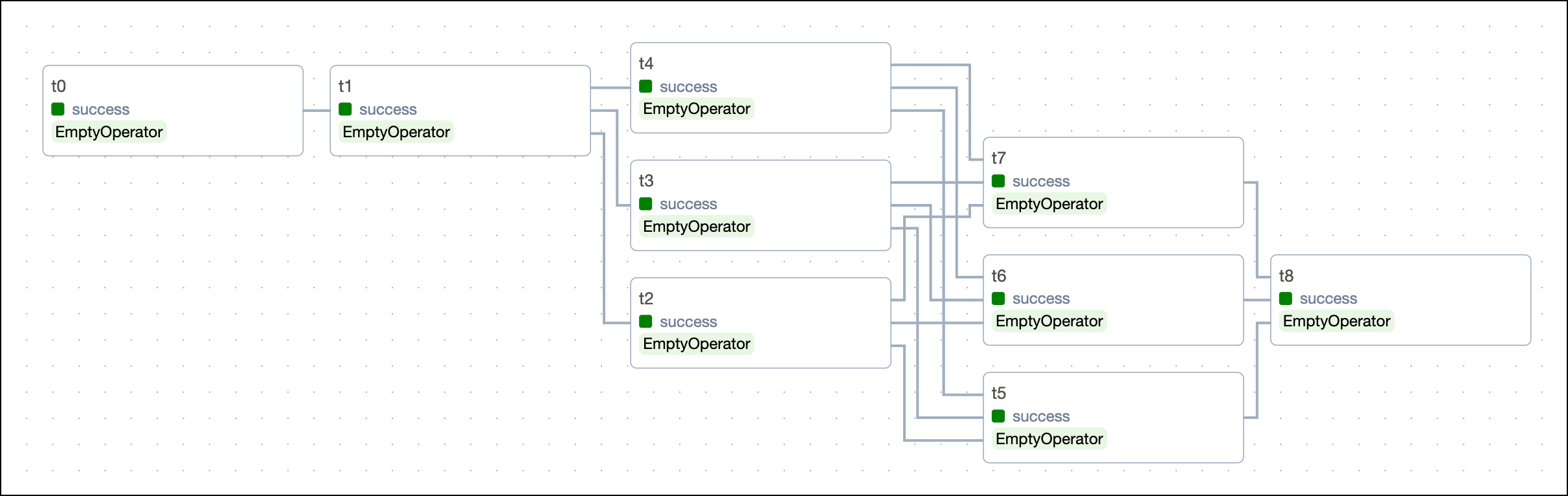The `chain_linear()` function can accept lists of any length in any order. For example, the following arguments are valid:

``chain_linear([t0, t1], [t2, t3, t4])``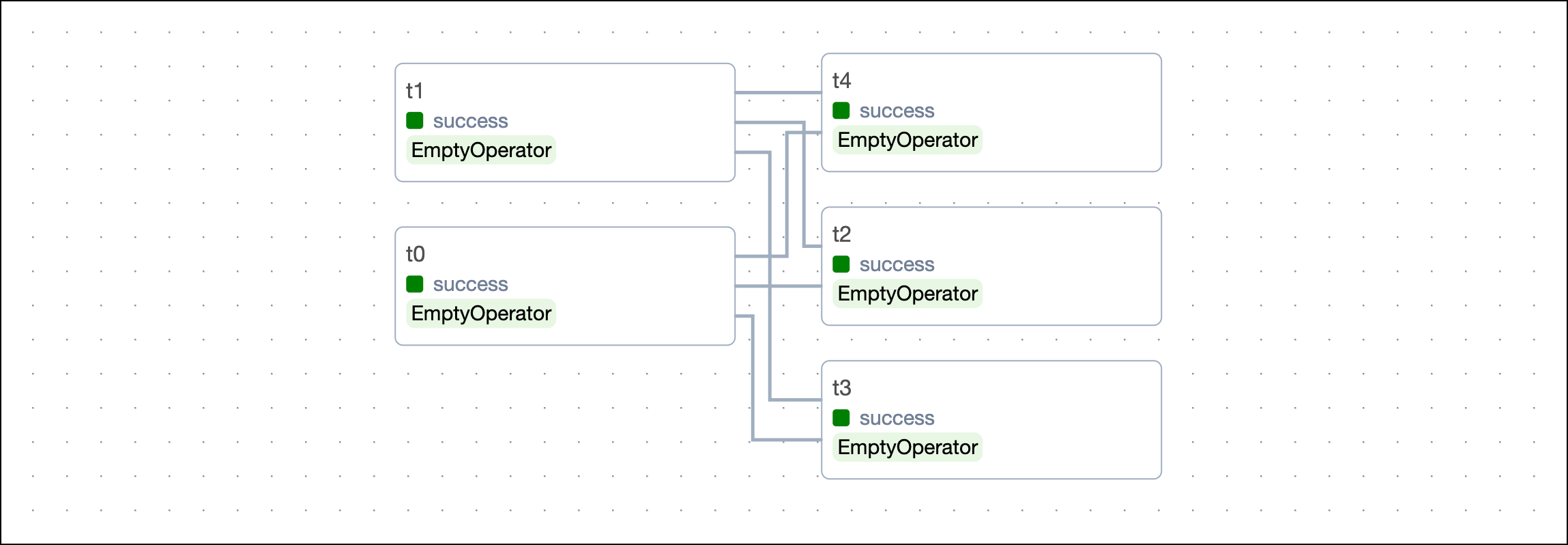## Dependencies in dynamic task mapping​

Dependencies for dynamically mapped tasks can be set in the same way as regular tasks. Note that when using the default trigger rule `all_success`, all mapped task instances need to be successful for the downstream task to run. For the purpose of trigger rules, mapped task instances behave like a set of parallel upstream tasks.

``start=EmptyOperator(task_id="start")@task def multiply(x,y):    return x*ymultiply_obj = multiply.partial(x=2).expand(y=[1,2,3])# end will only run if all mapped task instances of the multiply task are successfulend = EmptyOperator(task_id="end")start >> multiply_obj >> end# all of the following ways of setting dependencies are valid# multiply_obj.set_downstream(end)# end.set_upstream(multiply_obj)# chain(start, multiply_obj, end)``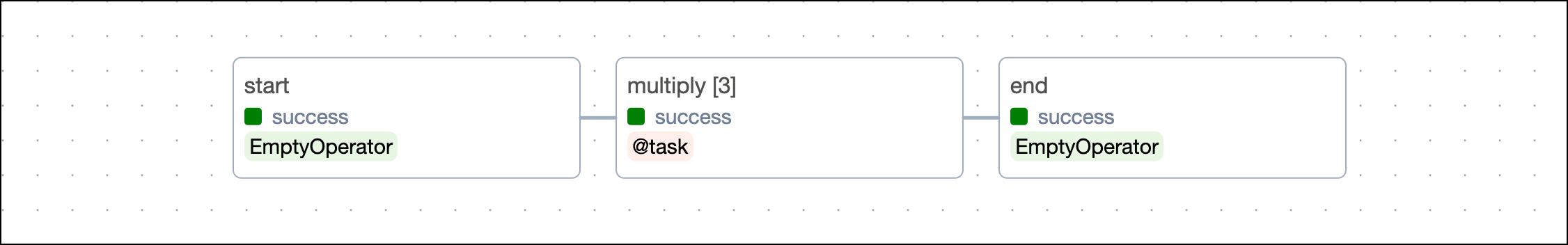Task groups logically group tasks in the Airflow UI and can be mapped dynamically. This section will explain how to set dependencies between task groups.

Dependencies can be set both inside and outside of a task group. For example, in the following DAG code there is a start task, a task group with two dependent tasks, and an end task. All of these tasks need to happen sequentially. The dependencies between the two tasks in the task group are set within the task group's context (`t1 >> t2`). The dependencies between the task group and the start and end tasks are set within the DAG's context (`t0 >> tg1() >> t3`).

``t0 = EmptyOperator(task_id="start")# Start task group definition@task_group(    group_id="group1")def tg1():    t1 = EmptyOperator(task_id="task1")    t2 = EmptyOperator(task_id="task2")    t1 >> t2# End task group definitiont3 = EmptyOperator(task_id="end")# Set task group's (tg1) dependenciest0 >> tg1() >> t3``

This image shows the resulting DAG: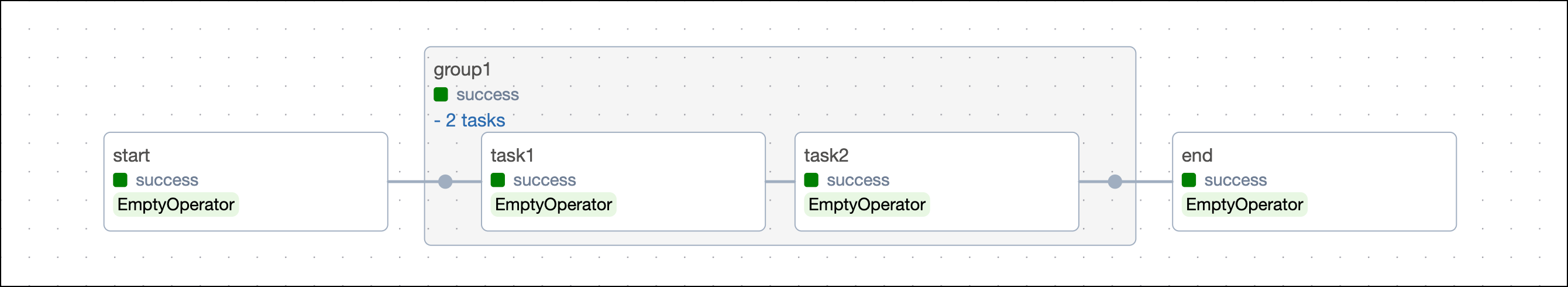The image below shows types of dependencies that can be set between tasks and task groups. You can find the code that created this DAG in a GitHub repository both for the TaskFlow API and traditional version.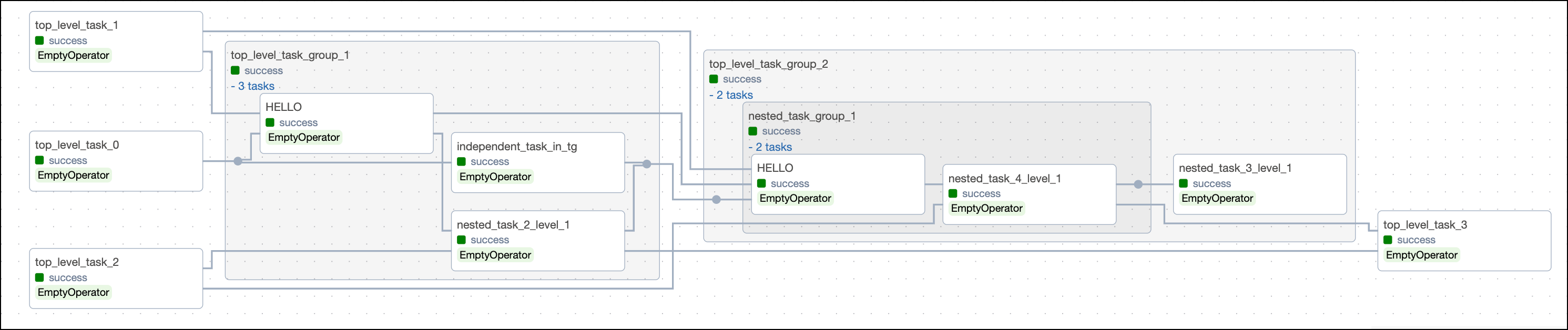The TaskFlow API `@task` decorator allows you to easily turn Python functions into Airflow tasks.

If your DAG has several tasks that are defined with the `@task` decorator and use each other's output, you can leverage inferred dependencies via the TaskFlow API. For example, in the following DAG there are two dependent tasks, `get_a_cat_fact` and `print_the_cat_fact`. To set the dependencies, you pass the called function of the upstream task as a positional argument to the downstream task (`print_the_cat_fact(get_a_cat_fact())`):

``from airflow.decorators import dag, taskfrom datetime import datetimeimport requestsimport jsonurl = "http://catfact.ninja/fact"default_args = {"start_date": datetime(2021, 1, 1)}@dag(schedule="@daily", default_args=default_args, catchup=False)def xcom_taskflow_dag():    @task    def get_a_cat_fact():        """        Gets a cat fact from the CatFacts API        """        res = requests.get(url)        return {"cat_fact": json.loads(res.text)["fact"]}    @task    def print_the_cat_fact(cat_fact: str):        """        Prints the cat fact        """        print("Cat fact for today:", cat_fact)        # run some further cat analysis here    # Invoke functions to create tasks and define dependencies    print_the_cat_fact(get_a_cat_fact())xcom_taskflow_dag()``

This image shows the resulting DAG: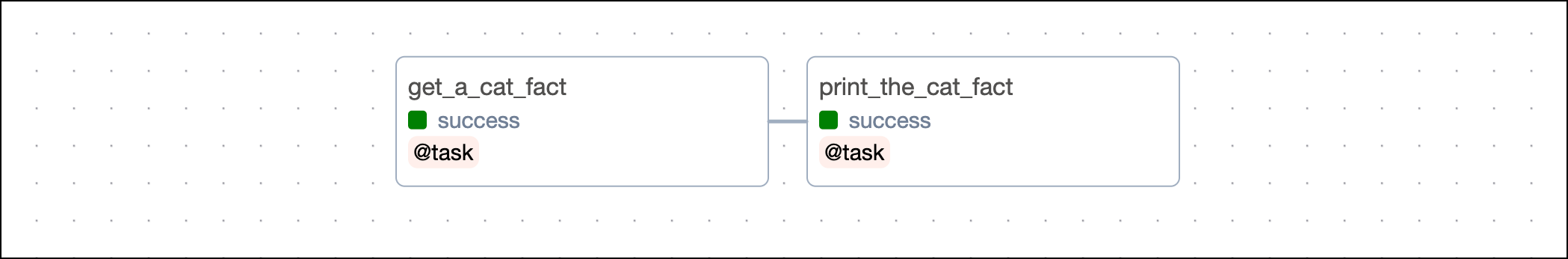Note that you can also assign the called function to an object and then pass that object to the downstream task. This way of defining dependencies is often easier to read and allows you to set the same task as an upstream dependency to multiple other tasks.

``@task def get_num():    return 42@task def add_one(num):    return num + 1@taskdef add_two(num):    return num + 2num = get_num()add_one(num)add_two(num)``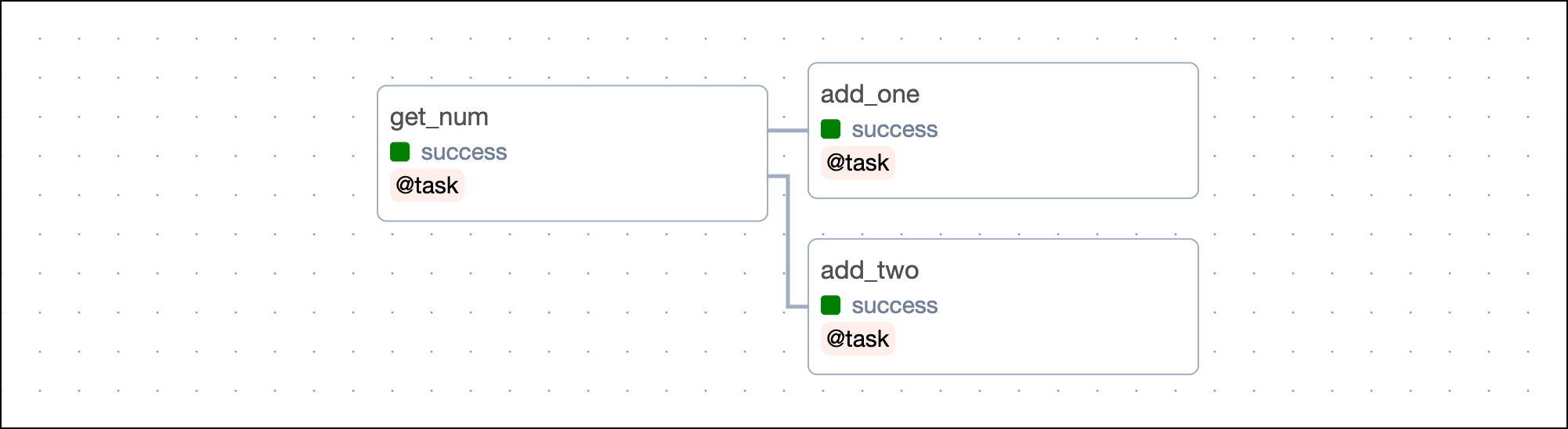If your DAG contains a mix of Python function tasks defined with decorators and tasks defined with traditional operators, you can set the dependencies by assigning the decorated task invocation to a variable and then defining the dependencies normally. For example, in the DAG below the `upload_data_to_s3` task is defined by the `@task` decorator and invoked with `upload_data = upload_data_to_s3(s3_bucket, test_s3_key)`. The `upload_data` variable is used in the last line to define dependencies.

``@dag(    start_date=datetime(2023, 1, 1),    schedule="@daily",    catchup=False)def sagemaker_model():    @task    def upload_data_to_s3(s3_bucket, test_s3_key):        """        Uploads validation data to S3 from /include/data         """        s3_hook = S3Hook(aws_conn_id="aws-sagemaker")        # Take string, upload to S3 using predefined method        s3_hook.load_file(            filename="include/data/test.csv",             key=test_s3_key,             bucket_name=s3_bucket,             replace=True        )    upload_data = upload_data_to_s3(s3_bucket, test_s3_key)    predict = SageMakerTransformOperator(        task_id="predict",        config=transform_config,        aws_conn_id="aws-sagemaker"    )    results_to_redshift = S3ToRedshiftOperator(        task_id="save_results",        aws_conn_id="aws-sagemaker",        s3_bucket=s3_bucket,        s3_key=output_s3_key,        schema="PUBLIC",        table="results",        copy_options=["csv"],    )    upload_data >> predict >> results_to_redshiftsagemaker_model()``

## Trigger rules​

When you set dependencies between tasks, the default Airflow behavior is to run a task only when all upstream tasks have succeeded. You can use trigger rules to change this default behavior.

The following options are available:

• `all_success`: (default) The task runs only when all upstream tasks have succeeded.
• `all_failed`: The task runs only when all upstream tasks are in a failed or upstream_failed state.
• `all_done`: The task runs once all upstream tasks are done with their execution.
• `all_skipped`: The task runs only when all upstream tasks have been skipped.
• `one_failed`: The task runs when at least one upstream task has failed.
• `one_success`: The task runs when at least one upstream task has succeeded.
• `one_done`: The task runs when at least one upstream task has either succeeded or failed.
• `none_failed`: The task runs only when all upstream tasks have succeeded or been skipped.
• `none_failed_min_one_success`: The task runs only when all upstream tasks have not failed or upstream_failed, and at least one upstream task has succeeded.
• `none_skipped`: The task runs only when no upstream task is in a skipped state.
• `always`: The task runs at any time.

### Branching and trigger rules​

One common scenario where you might need to implement trigger rules is if your DAG contains conditional logic such as branching. In these cases, `one_success` might be a more appropriate rule than `all_success`.

In the following example DAG there is a simple branch with a downstream task that needs to run if either of the branches are followed. With the `all_success` rule, the `end` task never runs because all but one of the `branch` tasks is always ignored and therefore doesn't have a success state. If you change the trigger rule to `one_success`, then the `end` task can run so long as one of the branches successfully completes.

``import randomfrom airflow.decorators import dag, taskfrom airflow.operators.empty import EmptyOperatorfrom datetime import datetimefrom airflow.utils.trigger_rule import TriggerRule@dag(start_date=datetime(2021, 1, 1), max_active_runs=1, schedule=None, catchup=False)def branching_dag():    # EmptyOperators to start and end the DAG    start = EmptyOperator(task_id="start")    end = EmptyOperator(task_id="end", trigger_rule=TriggerRule.ONE_SUCCESS)    # Branching task    @task.branch    def branching(**kwargs):        branches = ["branch_0", "branch_1", "branch_2"]        return random.choice(branches)    branching_task = branching()    start >> branching_task    # set dependencies    for i in range(0, 3):        d = EmptyOperator(task_id="branch_{0}".format(i))        branching_task >> d >> endbranching_dag()``

This image shows the resulting DAG: